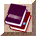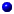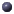##struct edp

Early drop parameters, supplied by userint bytes
true if queue in bytes, false if packetsint gentle
true to increases dropping probdouble max_p_inv
1/max_p, for max_p = maximum probint mean_pktsize
User supplieddouble ptc
Computed as a function of user supplied paramtersdouble q_w
queue weight given to cur q size sampleint setbit
true to set congestion indication bitdouble th_max
maximum threshold of average queue sizedouble th_min
minimum threshold of average queue sizeint wait
true for waiting between dropped packets

## Documentation

Early drop parameters, supplied by userint mean_pktsize
User supplied. avg pkt size, linked into Tclint bytes
true if queue in bytes, false if packetsint wait
true for waiting between dropped packetsint setbit
true to set congestion indication bitint gentle
true to increases dropping prob. slowly * when ave queue exceeds maxthresh.double th_min
minimum threshold of average queue sizedouble th_max
maximum threshold of average queue sizedouble max_p_inv
1/max_p, for max_p = maximum prob.double q_w
queue weight given to cur q size sampledouble ptc
Computed as a function of user supplied paramters. packet time constant in packets/second